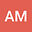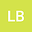Numerical approximations of time fractional multi dimensional Burger's equation using time-space pseudospectral method
••• AVINASH MITTAL,
• Lokendra Balyan
AVINASH MITTAL
Indian Institute of Information Technology Design and Manufacturing Jabalpur
Author ProfileLokendra Balyan
Indian Institute of Information Technology Design and Manufacturing Jabalpur
Author Profile## Abstract

In this paper, the authors approximate the solution of time fractional multi- dimensional Burger’s equation using the time-space Chebyshev pseudospectral method. Caputo fractional derivatives formula is used to illustrate the fractional derivatives matrix at CGL points. Using the Chebyshev fractional derivatives matrices the given problem is reduced to a system of nonlinear algebraic equations. These equations can be solved using Newton’s iterative method. Error analysis of the proposed method for the equation is presented. Model examples of time-fractional Burger’s equation are tested for a set of values of $\nu$, where $\nu$ represent the fractional order. For the proposed method, highly accurate numerical results are obtained which are compared with the analytical solution to confirm the accuracy and efficiency of the proposed method.